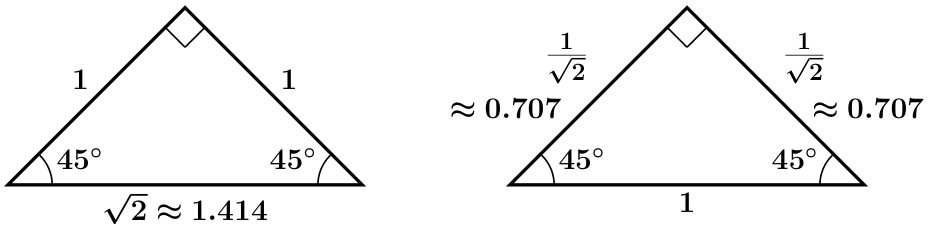# Tangent

Define tangent, and identify that the angle of reference for all of those tangent values.

Next, calculate tangent of all three 60 degree angles. What can you say about tangent values for angles in similar triangles?

Next compare the values of tan (30) and tan (60).

Repeat this process for tangent for the two 45 degree angles in the following triangles.How would you find a missing angle in a right triangle if you only know the 90 degree angle, but know 2 of the sides?  How might you use what you know about the tangent to find the other two angles.  Watch the video below and remember to return to this page by using the back arrow.

Inverse Trig Ratios (Soh-Cah-Toa)

Use inverse tangent to solve the following problem:Find the measure of $$\angle{X}$$ and $$\angle{Y}$$.

Did you find that $$\angle{X} = 22.6˚$$ and $$\angle{Y} = 67.4˚$$ ?